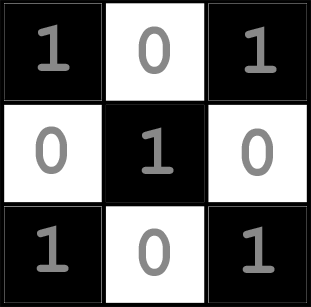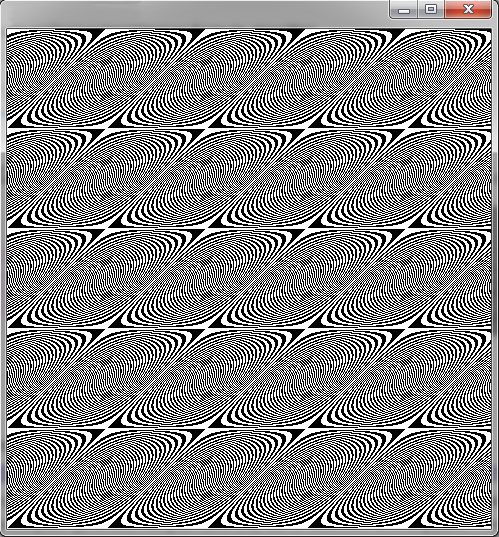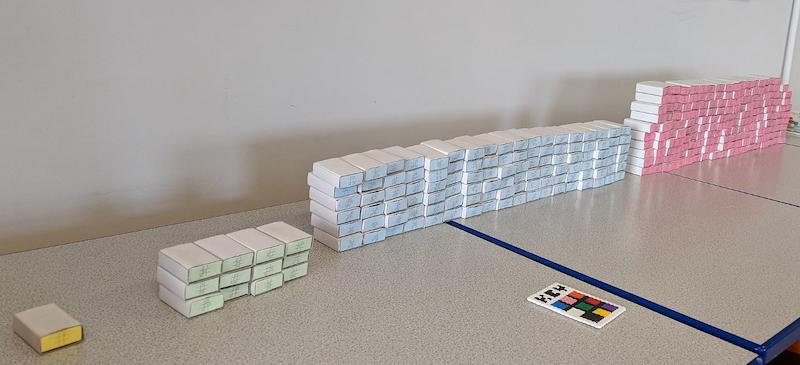Technical Article

# Learning About Algorithms One Step at a Time

August 04, 2021 by Greg Schmidt

## Algorithms can involve complicated programming. However, they didn’t start out that way. This article dives into basic algorithms like sorting and image generation.

These days, we often hear about algorithms. An algorithm may present online content such as a video of interest or a friend suggestion. A phone can recognize its owner. Automobile engines are tightly monitored and regulated by a computer. Driverless vehicles are being developed. All of these tasks are governed by algorithms.

But what exactly is an algorithm? Simply put, an algorithm is any procedure composed of a sequence of steps that, when carried out (i.e., “executed”), produces a desired result.

For instance, the recipe for baking a cake involves adding specific amounts of flour and sugar, placing it in the oven for a given amount of time, and then adding the frosting. The recipe is usually a set of printed instructions, and the baker is the agent that executes those instructions. Another example is crossing the street. The steps might include walking to the curb, looking right and waiting until no cars are approaching, looking left and again make sure that no cars are approaching, then proceeding to the other side. These represent legitimate algorithms.

Prior to the computer age, algorithms were typically executed by humans. During the 1940s and 1950s, the term "computer" often referred to a room filled with a group of women manually carrying out a sequence of calculations for such things as ballistic tables (figure 1). However, we will focus this discussion on including algorithms intended to be executed by a digital computer.##### Figure 1. Human “computers” manually performing an algorithmic computation. Image used courtesy of Computer History Museum

Fun Fact: The term "algorithm" originated from a 9th-century Persian scholar by the name of Musa al-Khwarizmi. He was an astronomer, geographer, and mathematician known for his contributions to algebra.

### Characteristics of an Algorithm

The result, or output, of an algorithm is somewhat arbitrary. It may be a single number resulting from a set of calculations, but it can also be a decision, such as “turn left” or "increase the fan speed."

An algorithm should conform to some basic properties:

• Executing the algorithm should produce a result. That may seem obvious, but it's not always clear if a complex set of steps will ever "terminate" with a result.
• The algorithm should produce a timely result. For example, a guidance algorithm for a space vehicle must produce results within microsecond intervals to be useful, whereas a weather simulation may acceptably produce results in a matter of hours. The size of the problem can affect timeliness. As the size of the problem grows, so does the amount of time taken to solve it.
• Executing the algorithm should not exceed the computer’s storage capacity. An algorithm may be designed to accept a range of problem sizes. Generally, the larger the problem, the more computer memory is required.
• For various reasons, not all algorithms produce exact results. For example, obtaining an exact result may be computationally impractical or even impossible for a very large problem. An approximation may be sufficient, and the acceptable limits of that approximation should be well understood.

The application of algorithms is vast, so we’ll examine just a few varied applications in a bit more detail.

### Sorting Algorithms

Let's begin with a simple sorting example. Suppose we have three numbers, and we want to sort them in ascending order. We’ll assume that somehow the numbers are stored in computer memory storage cells, and we can refer to them as "cell A," "cell B," and "cell C."

The following steps are guaranteed to work no matter the starting order:

1) If the contents of cell A is greater than the contents of cell B, then exchange the contents of the two cells.
2) If the contents of cell B is greater than the contents of cell C, then exchange the contents of the two cells.
3) Repeat steps 1 and 2.
4) Done.

To make things challenging, we'll start with an example list in descending order.

 3,2,1 (initial sequence in descending order) 2,3,1 (exchange cell A and cell B) 2,1,3 (exchange cell B and cell C) 1,2,3 (exchange cell A and cell B) The final sequence is now in ascending order.

Note that if we were to replace "greater than" with "less than" in the above sequence of steps, the algorithm will sort the list in descending order.

 1,2,3 (initial sequence in ascending order) 2,1,3, (exchange cell A and cell B) 2,3,1 (exchange cell B and cell C) 3,2,1 (exchange cell A and cell B) The final sequence is now in descending order.

Since numbers tend to “bubble up” from right to left, this particular algorithm is called “bubblesort.”

Hopefully, the concept of an algorithm is becoming a bit clearer, but how useful is a sorting algorithm that can only deal with three numbers? Actually, the above algorithm can easily be generalized to work with any amount of numbers. Bubblesort is not considered an efficient algorithm, and there are far better sorting algorithms, but an advantage of bubble sort is that it is easy to understand and works well on small lists of numbers.

### Image Generation Algorithms

Algorithms are commonly used to produce new images or process existing images. For example, certain algorithms can sharpen a fuzzy image, blur it, find the edges of objects, convert a color image to grayscale, and sophisticated algorithms can convert a black and white image to color.

An image is merely represented as a list of numbers stored in computer memory. Each pixel in the image is assigned a value based on its color. If the image is strictly black and white, then a 0 can represent the color white, and the number 1 can represent black.##### Figure 2. An image representing the numbers associated with image colors.

It is often the case that a very simple algorithm can produce surprisingly complex or unexpected results. Suppose we have the following equation, which determines whether or not any pixel in a  500 x 500 rectangular image should be painted as black or white.

(X * X + X*Y) / 30

X represents the value of the X coordinate of the pixel, and Y represents the Y coordinate. We divide by 30 (an experimentally determined number used to scale the result). If the number resulting from the above equation is even, we draw a black pixel at that location; otherwise, we draw a white pixel. We do this for all pixel locations as per their (X, Y) coordinates. That’s all there is to it! In figure 3, we see a rather unexpected image, certainly one that would be nearly impossible to predict from merely examining the above equation.##### Figure 3. An algorithmically generated graphic image.

Computer-generated color “fractal” images use more complex formulas to produce strikingly beautiful images (figure 4).### Machine Learning

Some algorithms can improve themselves. These are commonly referred to as “learning algorithms.” In the early 1960s, Donald Michie developed an algorithm called MENACE that could learn to play tic-tac-toe after playing many simulated games. The algorithm begins with no knowledge of the game, plays randomly at first, and then gradually learns the optimal strategy.

Interestingly, the first version of MENACE was not a computer program, but instead a collection of matchboxes. Michie was able to distill each possible board state (taking into consideration rotations and reflections of the board) into 304 patterns, each represented by a distinct matchbox. Each matchbox is labeled with a unique pattern of the tic-tac-toe board configuration (figure 5). In addition, each box begins with an equal number of beads of different colors. Each color represents a possible move that can be made from that particular game state.##### Figure 5. A recreation of MENACE built by Matthew Scroggs. Image used courtesy of Matthew Scroggs

The machine “plays” a move by first having the user select the matchbox that corresponds to the current game board, shakes it, and then randomly removes a bead from the box. The color of the bead determines the move. The machine must begin the game, so the matchbox that represents the empty board is initially selected. For example, if the bead removed is white and the color white represents the center square, the machine plays the center square.

The opponent then makes their move, and the process of selecting a bead from the matchbox is repeated using the revised board state. The game is played until there is a win, loss, or draw. Once the game has ended, the beads in all of the opened matchboxes are updated as follows:

• If the machine won – The removed bead, along with three additional beads of the same color, is added to the matchbox (major reward).
• If the machine lost – The bead removed during play is not returned to the matchbox (punishment).
• If there was a draw – The removed bead, along with another bead of the same color, are added to the matchbox (minor reward).

Initially, the machine plays poorly, but after playing many games and updating the matchbox with different color beads, the machine learns to play perfect tic-tac-toe.

MENACE was later programmed on a computer, and eventually, numerous advanced computer programs started using a similar, more sophisticated version of the algorithm. The general algorithm is referred to as “reinforcement learning.” Reinforcement learning has been applied to more complex games such as Chess and Go, and these algorithms are now capable of beating the most skilled human players.

We examined a few algorithms from different disciplines, though we only skimmed the surface of possibilities. Hopefully, this article provided you with a glimpse of insight into the realm of algorithms that have become so prevalent in our world.